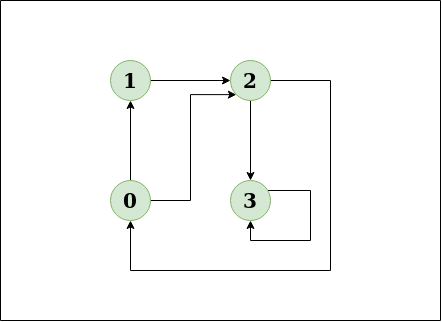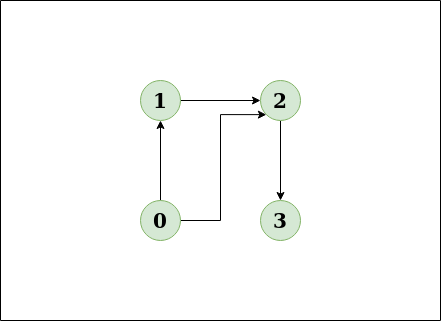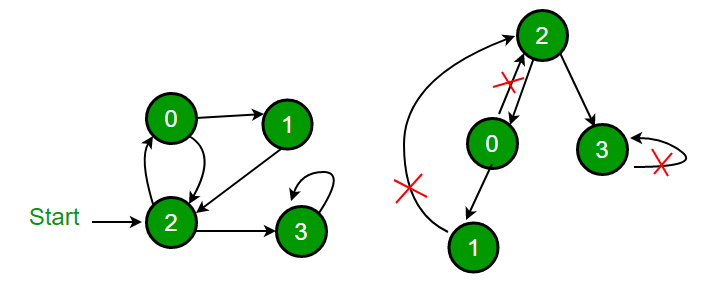Related Articles

# Detect Cycle in a directed graph using colors

• Difficulty Level : Medium
• Last Updated : 02 Jul, 2021

Given a directed graph, check whether the graph contains a cycle or not. Your function should return true if the given graph contains at least one cycle, else return false.

Example:

Input: n = 4, e = 6
0 -> 1, 0 -> 2, 1 -> 2, 2 -> 0, 2 -> 3, 3 -> 3
Output: Yes
Explanation:This diagram clearly shows a cycle 0 -> 2 -> 0.

Input:n = 4, e = 3
0 -> 1, 0 -> 2, 1 -> 2, 2 -> 3
Output:No
Explanation:This diagram clearly shows no cycle.

Solution
Approach: Depth First Traversal can be used to detect cycle in a Graph. DFS for a connected graph produces a tree. There is a cycle in a graph only if there is a back edge present in the graph. A back edge is an edge that is from a node to itself (selfloop) or one of its ancestor in the tree produced by DFS. In the following graph, there are 3 back edges, marked with cross sign. It can be observed that these 3 back edges indicate 3 cycles present in the graph.For a disconnected graph, we get the DFS forest as output. To detect cycle, we can check for cycle in individual trees by checking back edges.

Image Source: http://www.cs.yale.edu/homes/aspnes/pinewiki/DepthFirstSearch.html
In the previous post, we have discussed a solution that stores visited vertices in a separate array which stores vertices of the current recursion call stack.

In this post, a different solution is discussed. The solution is from CLRS book. The idea is to do DFS of a given graph and while doing traversal, assign one of the below three colours to every vertex.

WHITE : Vertex is not processed yet. Initially, all vertices are WHITE.
GRAY: Vertex is being processed (DFS for this vertex has started, but not finished which means that all descendants (in DFS tree) of this vertex are not processed yet (or this vertex is in the function call stack)
BLACK : Vertex and all its descendants are processed. While doing DFS, if an edge is encountered from current vertex to a GRAY vertex, then this edge is back edge and hence there is a cycle.

Algorithm:

1. Create a recursive function that takes the edge and color array (this can be also kept as a global variable)
2. Mark the current node as GREY.
3. Traverse all the adjacent nodes and if any node is marked GREY then return true as a loop is bound to exist.
4. If any adjacent vertex is WHITE then call the recursive function for that node. If the function returns true. Return true.
5. If no adjacent node is grey or has not returned true then mark the current Node as BLACK and return false.

Implementation:

## C++

 `// A DFS based approach to find if there is a cycle``// in a directed graph.  This approach strictly follows``// the algorithm given in CLRS book.``#include ``using` `namespace` `std;` `enum` `Color {WHITE, GRAY, BLACK};` `// Graph class represents a directed graph using``// adjacency list representation``class` `Graph``{``    ``int` `V; ``// No. of vertices``    ``list<``int``>* adj; ``// adjacency lists` `    ``// DFS traversal of the vertices reachable from v``    ``bool` `DFSUtil(``int` `v, ``int` `color[]);``public``:``    ``Graph(``int` `V);  ``// Constructor` `    ``// function to add an edge to graph``    ``void` `addEdge(``int` `v, ``int` `w);` `    ``bool` `isCyclic();``};` `// Constructor``Graph::Graph(``int` `V)``{``    ``this``->V = V;``    ``adj = ``new` `list<``int``>[V];``}` `// Utility function to add an edge``void` `Graph::addEdge(``int` `v, ``int` `w)``{``    ``adj[v].push_back(w); ``// Add w to v's list.``}` `// Recursive function to find if there is back edge``// in DFS subtree tree rooted with 'u'``bool` `Graph::DFSUtil(``int` `u, ``int` `color[])``{``    ``// GRAY :  This vertex is being processed (DFS``    ``//         for this vertex has started, but not``    ``//         ended (or this vertex is in function``    ``//         call stack)``    ``color[u] = GRAY;` `    ``// Iterate through all adjacent vertices``    ``list<``int``>::iterator i;``    ``for` `(i = adj[u].begin(); i != adj[u].end(); ++i)``    ``{``        ``int` `v = *i;  ``// An adjacent of u` `        ``// If there is``        ``if` `(color[v] == GRAY)``          ``return` `true``;` `        ``// If v is not processed and there is a back``        ``// edge in subtree rooted with v``        ``if` `(color[v] == WHITE && DFSUtil(v, color))``          ``return` `true``;``    ``}` `    ``// Mark this vertex as processed``    ``color[u] = BLACK;` `    ``return` `false``;``}` `// Returns true if there is a cycle in graph``bool` `Graph::isCyclic()``{``    ``// Initialize color of all vertices as WHITE``    ``int` `*color = ``new` `int``[V];``    ``for` `(``int` `i = 0; i < V; i++)``        ``color[i] = WHITE;` `    ``// Do a DFS traversal beginning with all``    ``// vertices``    ``for` `(``int` `i = 0; i < V; i++)``        ``if` `(color[i] == WHITE)``           ``if` `(DFSUtil(i, color) == ``true``)``              ``return` `true``;` `    ``return` `false``;``}` `// Driver code to test above``int` `main()``{``    ``// Create a graph given in the above diagram``    ``Graph g(4);``    ``g.addEdge(0, 1);``    ``g.addEdge(0, 2);``    ``g.addEdge(1, 2);``    ``g.addEdge(2, 0);``    ``g.addEdge(2, 3);``    ``g.addEdge(3, 3);` `    ``if` `(g.isCyclic())``        ``cout << ``"Graph contains cycle"``;``    ``else``        ``cout << ``"Graph doesn't contain cycle"``;` `    ``return` `0;``}`

## Java

 `import` `java.io.*;``import` `java.util.*;` `class` `GFG``{` `    ``// A DFS based approach to find if there is a cycle``    ``// in a directed graph. This approach strictly follows``    ``// the algorithm given in CLRS book.``    ``static` `int` `WHITE = ``0``, GRAY = ``1``, BLACK = ``2``;` `    ``// Graph class represents a directed graph using``    ``// adjacency list representation``    ``static` `class` `Graph``    ``{``            ``int` `V;``            ``LinkedList[] adjList;``            ` `            ``// Constructor``            ``Graph(``int` `ver)``            ``{``                ``V = ver;``                ``adjList = ``new` `LinkedList[V];``                ``for` `(``int` `i = ``0``; i < V; i++)``                    ``adjList[i] = ``new` `LinkedList<>();``            ``}``    ``}` `    ``// Utility function to add an edge``    ``static` `void` `addEdge(Graph g, ``int` `u, ``int` `v)``    ``{``            ``g.adjList[u].add(v); ``// Add v to u's list.``    ``}` `    ``// Recursive function to find if there is back edge``    ``// in DFS subtree tree rooted with 'u'``    ``static` `boolean` `DFSUtil(Graph g, ``int` `u, ``int``[] color)``    ``{``            ``// GRAY : This vertex is being processed (DFS``            ``// for this vertex has started, but not``            ``// ended (or this vertex is in function``            ``// call stack)``            ``color[u] = GRAY;``            ` `            ``// Iterate through all adjacent vertices``            ``for` `(Integer in : g.adjList[u])``            ``{``                ``// If there is``                ``if` `(color[in] == GRAY)``                    ``return` `true``;` `                ``// If v is not processed and there is a back``                ``// edge in subtree rooted with v``                ``if` `(color[in] == WHITE && DFSUtil(g, in, color) == ``true``)``                    ``return` `true``;``            ``}` `            ``// Mark this vertex as processed``            ``color[u] = BLACK;``            ``return` `false``;``    ``}` `    ``// Returns true if there is a cycle in graph``    ``static` `boolean` `isCyclic(Graph g)``    ``{``            ``// Initialize color of all vertices as WHITE``            ``int``[] color = ``new` `int``[g.V];``            ``for` `(``int` `i = ``0``; i < g.V; i++)``            ``{``                ``color[i] = WHITE;``            ``}` `            ``// Do a DFS traversal beginning with all``            ``// vertices``            ``for` `(``int` `i = ``0``; i < g.V; i++)``            ``{``                ``if` `(color[i] == WHITE)``                ``{``                    ``if``(DFSUtil(g, i, color) == ``true``)``                        ``return` `true``;``                ``}``            ``}``            ``return` `false``;` `    ``}` `    ``// Driver code to test above``    ``public` `static` `void` `main(String args[])``    ``{``            ``// Create a graph given in the above diagram``            ``Graph g = ``new` `Graph(``4``);``            ``addEdge(g, ``0``, ``1``);``            ``addEdge(g, ``0``, ``2``);``            ``addEdge(g, ``1``, ``2``);``            ``addEdge(g, ``2``, ``0``);``            ``addEdge(g, ``2``, ``3``);``            ``addEdge(g, ``3``, ``3``);``            ``if` `(isCyclic(g))``                ``System.out.println(``"Graph contains cycle"``);``            ``else``                ``System.out.println(``"Graph doesn't contain cycle"``);``    ``}``}` `// This code is contributed by rachana soma`

## Python

 `# Python program to deetect cycle in``# a directed graph` `from` `collections ``import` `defaultdict` `class` `Graph():``    ``def` `__init__(``self``, V):``        ``self``.V ``=` `V``        ``self``.graph ``=` `defaultdict(``list``)` `    ``def` `addEdge(``self``, u, v):``        ``self``.graph[u].append(v)` `    ``def` `DFSUtil(``self``, u, color):``        ``# GRAY :  This vertex is being processed (DFS``        ``#         for this vertex has started, but not``        ``#         ended (or this vertex is in function``        ``#         call stack)``        ``color[u] ``=` `"GRAY"` `        ``for` `v ``in` `self``.graph[u]:` `            ``if` `color[v] ``=``=` `"GRAY"``:``                ``return` `True` `            ``if` `color[v] ``=``=` `"WHITE"` `and` `self``.DFSUtil(v, color) ``=``=` `True``:``                ``return` `True` `        ``color[u] ``=` `"BLACK"``        ``return` `False` `    ``def` `isCyclic(``self``):``        ``color ``=` `[``"WHITE"``] ``*` `self``.V` `        ``for` `i ``in` `range``(``self``.V):``            ``if` `color[i] ``=``=` `"WHITE"``:``                ``if` `self``.DFSUtil(i, color) ``=``=` `True``:``                    ``return` `True``        ``return` `False` `# Driver program to test above functions` `g ``=` `Graph(``4``)``g.addEdge(``0``, ``1``)``g.addEdge(``0``, ``2``)``g.addEdge(``1``, ``2``)``g.addEdge(``2``, ``0``)``g.addEdge(``2``, ``3``)``g.addEdge(``3``, ``3``)``print` `"Graph contains cycle"` `if` `g.isCyclic() ``=``=` `True``\``                             ``else` `"Graph doesn't contain cycle"``                             ` `# This program is contributed by Divyanshu Mehta                            `

## C#

 `// A C# program to detect cycle in``// an undirected graph using BFS.``using` `System;``using` `System.Collections.Generic;` `class` `GFG``{` `    ``// A DFS based approach to find if``    ``// there is a cycle in a directed graph.``    ``// This approach strictly follows the``    ``// algorithm given in CLRS book.``    ``static` `int` `WHITE = 0, GRAY = 1, BLACK = 2;` `    ``// Graph class represents a directed graph``    ``// using adjacency list representation``    ``public` `class` `Graph``    ``{``        ``public` `int` `V;``        ``public` `List<``int``>[] adjList;``        ` `        ``// Constructor``        ``public` `Graph(``int` `ver)``        ``{``            ``V = ver;``            ``adjList = ``new` `List<``int``>[V];``            ``for` `(``int` `i = 0; i < V; i++)``                ``adjList[i] = ``new` `List<``int``>();``        ``}``    ``}` `    ``// Utility function to add an edge``    ``static` `void` `addEdge(Graph g, ``int` `u, ``int` `v)``    ``{``        ``g.adjList[u].Add(v); ``// Add v to u's list.``    ``}` `    ``// Recursive function to find if there is back edge``    ``// in DFS subtree tree rooted with 'u'``    ``static` `bool` `DFSUtil(Graph g, ``int` `u, ``int``[] color)``    ``{``        ``// GRAY : This vertex is being processed (DFS``        ``// for this vertex has started, but not``        ``// ended (or this vertex is in function``        ``// call stack)``        ``color[u] = GRAY;``        ` `        ``// Iterate through all adjacent vertices``        ``foreach` `(``int` `iN ``in` `g.adjList[u])``        ``{``            ``// If there is``            ``if` `(color[iN] == GRAY)``                ``return` `true``;` `            ``// If v is not processed and there is a back``            ``// edge in subtree rooted with v``            ``if` `(color[iN] == WHITE &&``                ``DFSUtil(g, iN, color) == ``true``)``                ``return` `true``;``        ``}` `        ``// Mark this vertex as processed``        ``color[u] = BLACK;``        ``return` `false``;``    ``}``    ` `    ``// Returns true if there is a cycle in graph``    ``static` `bool` `isCyclic(Graph g)``    ``{``        ``// Initialize color of all vertices as WHITE``        ``int``[] color = ``new` `int``[g.V];``        ``for` `(``int` `i = 0; i < g.V; i++)``        ``{``            ``color[i] = WHITE;``        ``}` `        ``// Do a DFS traversal beginning with all``        ``// vertices``        ``for` `(``int` `i = 0; i < g.V; i++)``        ``{``            ``if` `(color[i] == WHITE)``            ``{``                ``if``(DFSUtil(g, i, color) == ``true``)``                    ``return` `true``;``            ``}``        ``}``        ``return` `false``;` `    ``}` `    ``// Driver Code``    ``public` `static` `void` `Main(String []args)``    ``{``        ``// Create a graph given in the above diagram``        ``Graph g = ``new` `Graph(4);``        ``addEdge(g, 0, 1);``        ``addEdge(g, 0, 2);``        ``addEdge(g, 1, 2);``        ``addEdge(g, 2, 0);``        ``addEdge(g, 2, 3);``        ``addEdge(g, 3, 3);``        ``if` `(isCyclic(g))``            ``Console.WriteLine(``"Graph contains cycle"``);``        ``else``            ``Console.WriteLine(``"Graph doesn't contain cycle"``);``    ``}``}` `// This code is contributed by PrinciRaj1992`

## Javascript

 ``

Output:

`Graph contains cycle`

Complexity Analysis:

• Time complexity: O(V + E), where V is the number of vertices and E is the number of edges in the graph.
• Space Complexity :O(V).
Since an extra color array is needed of size V.